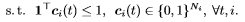Question for Constraint

(navro) #1

Hello,
Is there a way to express the following constraint on cvx subject to section?(Mark L. Stone) #2

You haven’t told us what c_i(t) means.

Nevertheless, variables, whether they be scalars, vectors, matrices, or n-d arrays, can be declared binary.

Here is a simplified situation to illustrate.

``````variable c(n) binary
sum(c) <= 1 % note that this is the same as ones(n,1)'*c <= 1
``````

I have no idea what all your indexing is, so I’ll let you deal with that.

(navro) #3

My cvx does not support binary variables.I get the SDTP3 does not support integer variables error.

c_i(t) is a coefficient subvector N_i x 1 and i want it to be 1 at most once cause i want to extract at most one column from the matrix he is assosiated with.

(Mark L. Stone) #4

You will need CVX and solver (Mosek or Gurobi) combination which supports binary and integer variables.

(Linmumuzf) #5

Hello, I would like to ask how to calculate the time complexity of KKT solution.Thank you very much.

(Erling D.Andersen) #6

This

may be relevant.

1 Like
(Linmumuzf) #7

Thank you, why can’t I get in this link?

(Mark L. Stone) #8

The link works for me. Perhaps you should try another browser? Or you have a site blocker?

(Erling D.Andersen) #9

Last time I was in China they blocked Google. So the link is not likely to work in China without using VPN.

1 Like